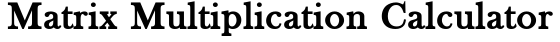##### Related Content

Programmers and Teachers:Nikkolas K.
Tutor and degreed Aerospace Engineer

# Matrix Multiplication CalculatorMatrix A: rows X columns
Matrix B: rows X columns
A =
B =

## Lesson on Matrix Multiplication

### Rules of Multiplication

Matrix multiplication adheres to different rules than matrix addition and subtraction. When multiplying a matrix by another matrix, the number of columns in the first matrix must match the number of rows in the second matrix. The first matrix may have any number of rows and the second matrix may have any number of columns.

The output matrix dimensions are defined by the dimensions of the input matrices. The formula for such is given as:
m×n x n×p → m×p
Where matrix A is of dimensions m×n, matrix B is of dimensions n×p, and matrix C is of dimensions m×p.

### How to Multiply a Matrix by Another Matrix

To multiply two matrices, we perform the dot product of rows and columns from matrix A and matrix B respectively. Consider each row of matrix A a vector with a number of components equal to the number of columns n. Then, consider each column of matrix B a vector with a number of components equal to the number of rows n.

Below is a literal example of using dot products to multiply a 3×2 matrix A by a 2×3 matrix B.

$$\left[\begin{matrix}a_{1i} & a_{1j}\\a_{2i} & a_{2j}\\a_{3i} & a_{3j}\end{matrix}\right] \times \left[\begin{matrix}b_{1i} & b_{2i} & b_{3i}\\b_{1j} & b_{2j} & b_{3j}\end{matrix}\right] = \left[\begin{matrix}a_{1} \cdot b_{1} & a_{1} \cdot b_{2} & a_{1} \cdot b_{3}\\a_{2} \cdot b_{1} & a_{2} \cdot b_{2} & a_{2} \cdot b_{3}\\a_{3} \cdot b_{1} & a_{3} \cdot b_{2} & a_{3} \cdot b_{3}\end{matrix}\right]$$

## How the Calculator Works

The calculator on this page is written in the programming language JavaScript (JS) and runs in your device’s internet browser JS engine, allowing for near-instant solutions at the click of a button. The code follows the same rules and steps as outlined in this lesson, with some additional steps and processes.

When the calculate button is clicked, a function is activated that contains several nested loops. The first loops create variables for each entry of matrix A and matrix B. Then, another set of loops create the output matrix C while performing the respective dot products of the entries from A and B. The final loop converts the output matrix into LaTeX, a math rendering language.

Once calculations finish, the LaTeX code of the output matrix is rendered in the answer area of the calculator. Sometimes the inputs cause an error or do not allow for a solution to be found. When these anomalies occur, an error statement is displayed instead of an answer.

Scroll to Top